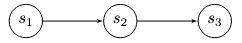# Transaction Chains#

A transaction chain is used for creating a multi-stage protocol whose code does not contain loops or if statements.

A transaction chain is created as follows:

## 1: Represent as N#

Represent an Ethereum contract’s execution using n sequential steps, where each step represents a transaction that modifies its state. The states before and after a transaction are a directed graph's start and end nodes, with the transaction as the edge joining them. As an example, a 3-stage contract, such as the ICO example of Section 4.3, is represented as:The states contain data and the code that was executed in the transaction.

## 2: State#

Hardwire state n’s code and data inside state n − 1’s code. Then require the code of state n − 1 to output a box containing state n’s code and data. An example is given in the following pseudocode:

out.propositionBytes == state_n_code &&
out.R4[Int].get == SELF.R4[Int].get // ensure data is propagated


The above code uses the field propositionBytes of a box, which contains the binary representation of its guard script as a collection of bytes.

## 3: Repeat#

Repeat Step 2 by replacing (n, n − 1) by (n − 1, n − 2) while n > 2

To avoid code size increase at each iteration, we ideally work with hashes, as in hash(out.propositionBytes) == state n code hash. However, for clarity of presentation, we will skip this optimization.

Next, we will look at Transaction Trees